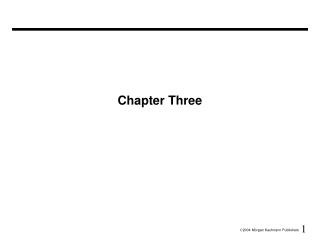DownloadDownload PresentationChapter Three

# Chapter Three

Télécharger la présentation## Chapter Three

- - - - - - - - - - - - - - - - - - - - - - - - - - - E N D - - - - - - - - - - - - - - - - - - - - - - - - - - -
##### Presentation Transcript

1. Chapter Three

2. Numbers • Bits are just bits (no inherent meaning) — conventions define relationship between bits and numbers • Binary numbers (base 2) 0000 0001 0010 0011 0100 0101 0110 0111 1000 1001... decimal: 0...2n-1 • Of course it gets more complicated: numbers are finite (overflow) fractions and real numbers negative numbers e.g., no MIPS subi instruction; addi can add a negative number • How do we represent negative numbers? i.e., which bit patterns will represent which numbers?

3. Possible Representations • Sign Magnitude: One's Complement Two's Complement 000 = +0 000 = +0 000 = +0 001 = +1 001 = +1 001 = +1 010 = +2 010 = +2 010 = +2 011 = +3 011 = +3 011 = +3 100 = -0 100 = -3 100 = -4 101 = -1 101 = -2 101 = -3 110 = -2 110 = -1 110 = -2 111 = -3 111 = -0 111 = -1 • Issues: balance, number of zeros, ease of operations • Which one is best? Why?

4. maxint minint MIPS • 32 bit signed numbers:0000 0000 0000 0000 0000 0000 0000 0000two = 0ten0000 0000 0000 0000 0000 0000 0000 0001two = + 1ten0000 0000 0000 0000 0000 0000 0000 0010two = + 2ten...0111 1111 1111 1111 1111 1111 1111 1110two = + 2,147,483,646ten0111 1111 1111 1111 1111 1111 1111 1111two = + 2,147,483,647ten1000 0000 0000 0000 0000 0000 0000 0000two = – 2,147,483,648ten1000 0000 0000 0000 0000 0000 0000 0001two = – 2,147,483,647ten1000 0000 0000 0000 0000 0000 0000 0010two = – 2,147,483,646ten...1111 1111 1111 1111 1111 1111 1111 1101two = – 3ten1111 1111 1111 1111 1111 1111 1111 1110two = – 2ten1111 1111 1111 1111 1111 1111 1111 1111two = – 1ten

5. Two's Complement Operations • Negating a two's complement number: invert all bits and add 1 • remember: “negate” and “invert” are quite different! • Converting n bit numbers into numbers with more than n bits: • MIPS 16 bit immediate gets converted to 32 bits for arithmetic • copy the most significant bit (the sign bit) into the other bits 0010 -> 0000 0010 1010 -> 1111 1010 • "sign extension" (lbu vs. lb)

6. Addition & Subtraction • Just like in grade school (carry/borrow 1s) 0111 0111 0110+ 0110 - 0110 - 0101 • Two's complement operations easy • subtraction using addition of negative numbers 0111 + 1010 • Overflow (result too large for finite computer word): • e.g., adding two n-bit numbers does not yield an n-bit number 0111 + 0001 note that overflow term is somewhat misleading, 1000 it does not mean a carry “overflowed”

7. Detecting Overflow • No overflow when adding a positive and a negative number • No overflow when signs are the same for subtraction • Overflow occurs when the value affects the sign: • overflow when adding two positives yields a negative • or, adding two negatives gives a positive • or, subtract a negative from a positive and get a negative • or, subtract a positive from a negative and get a positive • Consider the operations A + B, and A – B • Can overflow occur if B is 0 ? • Can overflow occur if A is 0 ?

8. Effects of Overflow • An exception (interrupt) occurs • Control jumps to predefined address for exception • Interrupted address is saved for possible resumption • Details based on software system / language • example: flight control vs. homework assignment • Don't always want to detect overflow — new MIPS instructions: addu, addiu, subu note: addiu still sign-extends! note: sltu, sltiu for unsigned comparisons

9. Multiplication • More complicated than addition • accomplished via shifting and addition • More time and more area • Let's look at 3 versions based on a gradeschool algorithm 0010 (multiplicand) __x_1011 (multiplier) • Negative numbers: convert and multiply • there are better techniques, we won’t look at them

10. Multiplication: Implementation Datapath Control

11. Final Version • Multiplier starts in right half of product What goes here?

12. Floating Point (a brief look) • We need a way to represent • numbers with fractions, e.g., 3.1416 • very small numbers, e.g., .000000001 • very large numbers, e.g., 3.15576 ´ 109 • Representation: • sign, exponent, significand: (–1)sign´ significand ´ 2exponent • more bits for significand gives more accuracy • more bits for exponent increases range • IEEE 754 floating point standard: • single precision: 8 bit exponent, 23 bit significand • double precision: 11 bit exponent, 52 bit significand

13. IEEE 754 floating-point standard • Leading “1” bit of significand is implicit • Exponent is “biased” to make sorting easier • all 0s is smallest exponent all 1s is largest • bias of 127 for single precision and 1023 for double precision • summary: (–1)sign´ (1+significand) ´ 2exponent – bias • Example: • decimal: -.75 = - ( ½ + ¼ ) • binary: -.11 = -1.1 x 2-1 • floating point: exponent = 126 = 01111110 • IEEE single precision: 10111111010000000000000000000000